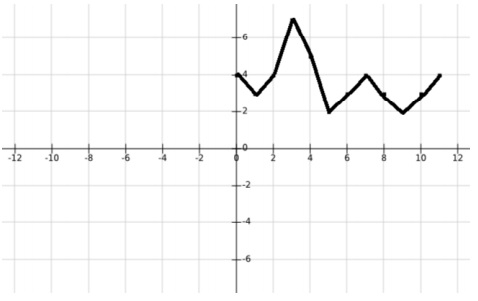# Finding all peaks and their positions in an array in JavaScript

JavascriptWeb DevelopmentFront End Technology

## Build Up

Suppose we have the following array in JavaScript −

const arr = [4, 3, 4, 7, 5, 2, 3, 4, 3, 2, 3, 4];

If we plot the points of this array on y-axis with each adjacent point being unit distance away on x-axis, the graph will look like this −This graph clearly shows that there exist two local maxima (peaks) in this array at index 3 and 7 with values 7 and 4 respectively.

## Problem

We are required to write a JavaScript function that takes in an array of integers, arr, as the first and the only argument.

Our function is supposed to return an object that contains two properties, maximas, and positions.

Both these properties will be arrays, and the maxima array will contain the value of local maximas in the array and the positions array will contain their corresponding indices.

For example, if the input to the function is −

Therefore, for the above array, the output should look like −

const output = {
maximas: [7, 4],
positions: [3, 7]
};

## Example

Following is the code −

Live Demo

const arr = [4, 3, 4, 7, 5, 2, 3, 4, 3, 2, 3, 4];
const findMaxima = (arr = []) => {
let positions = []
let maximas = []
for (let i = 1; i < arr.length - 1; i++) {
if (arr[i] > arr[i - 1]) {
if (arr[i] > arr[i + 1]) {
positions.push(i)
maximas.push(arr[i])
} else if (arr[i] === arr[i + 1]) {
let temp = i
while (arr[i] === arr[temp]) i++
if (arr[temp] > arr[i]) {
positions.push(temp)
maximas.push(arr[temp])
}
}
}
}
return { maximas, positions };
};
console.log(findMaxima(arr));

## Output

Then the output should be −

{ maximas: [ 7, 4 ], positions: [ 3, 7 ] }